# Application to linear models#### Everything You Need in One Place

Homework problems? Exam preparation? Trying to grasp a concept or just brushing up the basics? Our extensive help & practice library have got you covered.#### Learn and Practice With Ease

Our proven video lessons ease you through problems quickly, and you get tonnes of friendly practice on questions that trip students up on tests and finals.#### Instant and Unlimited Help

Our personalized learning platform enables you to instantly find the exact walkthrough to your specific type of question. Activate unlimited help now!Get the most by viewing this topic in your current grade. Pick your course now.

##### Intros
###### Lessons
1. Applications to Linear Models Overview:
2. Applying Least-Squares Problem to Economics
• Go from $Ax=b$ to $X\beta=y$
$X$→ design matrix
$\beta$→ parameter vector
$y$→ observation vector
3. Least-Squares Line
• Finding the best fit line
• Turning a system of equations into $X\beta =y$
• Using the normal equation $X^T X\beta=X^T y$
• Introduction of the residual vector
4. Least-Squares to Other Curves
• Finding the Best Fit Curve (not a line)
• Using the normal equation $X^T X\beta=X^T y$
5. Least-Squares to Multiple Regressions
• Multiple Regression → multivariable function
• Finding a Best Fit Plane
• Using the normal equation $X^T X\beta=X^T y$
##### Examples
###### Lessons
1. Finding the Least-Squares Line
Find the equation $y=\beta_0+\beta_1 x$ of the least-squares line that best fits the given data points:
$(0,1),(1,2),(2,3),(3,3)$
1. Finding the Least-Squares of Other Curves
Suppose the monthly costs of a product depend on seasonal fluctuations. A curve that approximates the cost is
$y= \beta _0 + \beta _1 x+ \beta _2 x^2 + \beta_3 \cos$ ($\frac{2 \pi x}{12}$)

Suppose you want to find a better approximation in the future by evaluating the residual errors in each data point. Let's assume the errors for each data point to be $\epsilon_1,\epsilon_2,\cdots,\epsilon_n$.
Give the design matrix, parameter vector, and residual vector for the model that leads to a least-squares fit for the equation above. Assume the data are $(x_1,y_1 ),\cdots,(x_n,y_n).$
1. An experiment gives the data points $(0,1) , (1,3) , (2, 4), (3, 5)$. Suppose we wish to approximate the data using the equation
$y=A+Bx^2$

First find the design matrix, observational vector, and unknown parameter vector. No need to find the residual vector. Then find the least-squares curve for the data.
1. Finding the Least Squares of Multiple Regressions
When examining a local model of terrain, we examine the data points to be $(1,1, 3), (2, 2, 5),$ and $(3, 1, 3)$. Suppose we wish to approximate the data using the equation
$y=\beta_0 u+\beta_1 v$

First find the design matrix, observational vector, and unknown parameter vector. No need to find the residual vector. Then find the least-squares curve for the data.
1. Proof Question Relating to Linear Models
Show that
$\lVert X \hat{\beta} \rVert^2=\beta^TX^Ty$
###### Free to Join!
StudyPug is a learning help platform covering math and science from grade 4 all the way to second year university. Our video tutorials, unlimited practice problems, and step-by-step explanations provide you or your child with all the help you need to master concepts. On top of that, it's fun - with achievements, customizable avatars, and awards to keep you motivated.
• #### Easily See Your ProgressWe track the progress you've made on a topic so you know what you've done. From the course view you can easily see what topics have what and the progress you've made on them. Fill the rings to completely master that section or mouse over the icon to see more details.
• #### Make Use of Our Learning Aids###### Practice Accuracy

Get quick access to the topic you're currently learning.

See how well your practice sessions are going over time.

Stay on track with our daily recommendations.

• #### Earn Achievements as You LearnMake the most of your time as you use StudyPug to help you achieve your goals. Earn fun little badges the more you watch, practice, and use our service.
• #### Create and Customize Your AvatarPlay with our fun little avatar builder to create and customize your own avatar on StudyPug. Choose your face, eye colour, hair colour and style, and background. Unlock more options the more you use StudyPug.
###### Topic Notes
In this section, we will apply least square problems to economics.

Instead of finding the least squares solution of $Ax=b$, we will be finding it for $X\beta =y$ where

$X$→ design matrix
$\beta$→ parameter vector
$y$→ observation vector

Least-Squares Line
Suppose we are given data points, and we want to find a line that best fits the data points. Let the best fit line be the linear equation
$y=\beta_0 + \beta_1 x$

And let the data points be $(x_1,y_1 ),(x_2,y_2 ),\cdots,,(x_n,y_n )$. The graph should look something like this: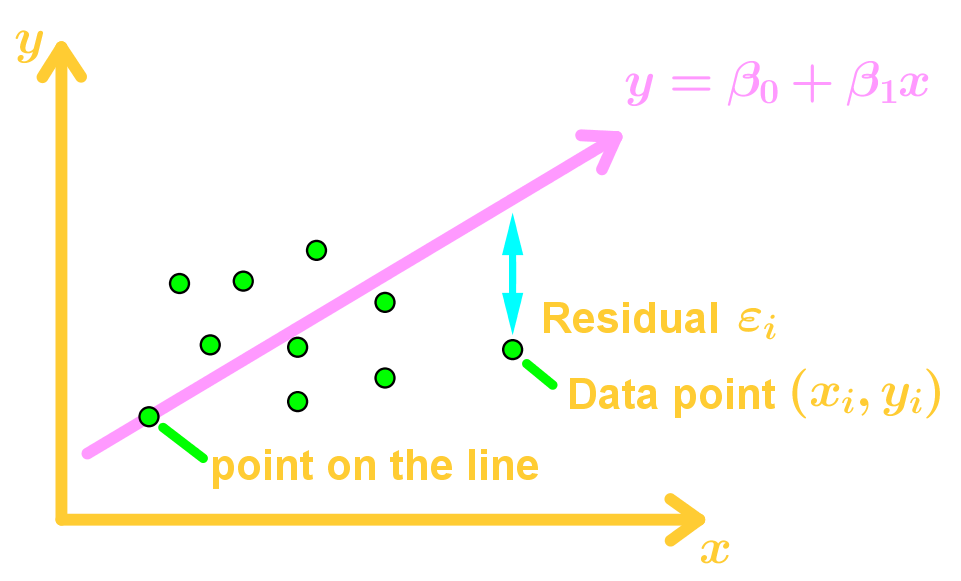Our goal is to determine the parameters $\beta_0$ and $\beta_1$. Let's say that each data point is on the line. Then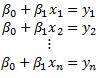This is a linear system which we can write this as: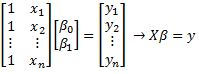Then the least squares solution to $X\beta=y$ will be $X^T X \beta =X^T y$.

General Linear Model
Since the data points are not actually on the line, then there are residual values. Those are also known as errors. So we introduce a vector called the residual vector $\epsilon$, where
$\epsilon = y - X\beta$
$y = X\beta + \epsilon$

Our goal is to minimize the length of $\epsilon$ (the error), so that $X\beta$ is approximately equal to $y$. This means we are finding a least-squares solution of $y=X\beta$ using $X^T X\beta=X^T y$.

Least-Squares of Other Curves
Let the data points be $(x_1,y_1 ),(x_2,y_2 ),\cdots,,(x_n,y_n )$ and we want to find the best fit using the function $y=\beta_0+\beta_1 x+\beta_2 x^2$, where $\beta_0,\beta_1,\beta_2$ are parameters. Technically we are using a best fit quadratic function instead of a line now.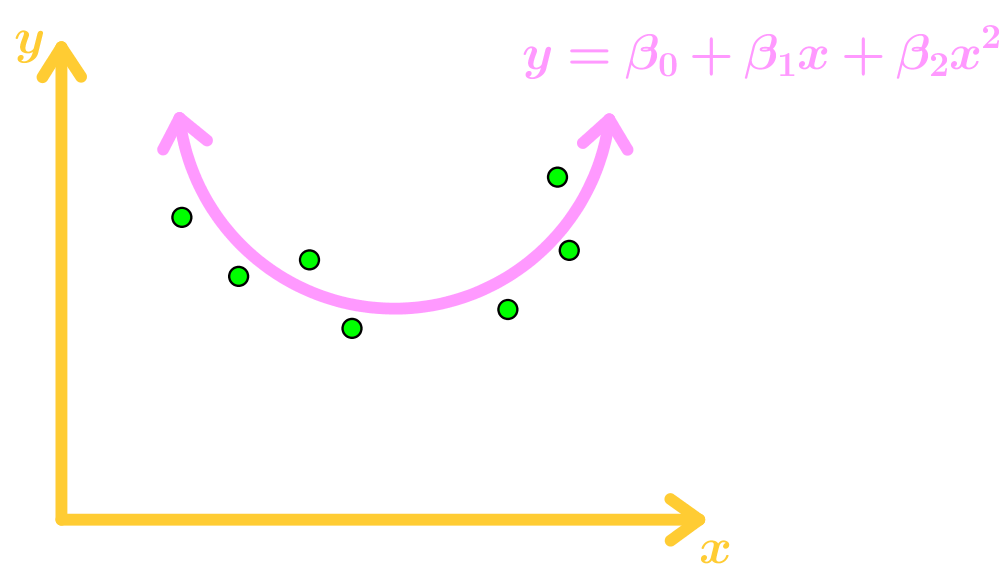Again, the data points don't actually lie on the function, so we add residue values $\epsilon_1,\epsilon_2,\cdots,\epsilon_n$ where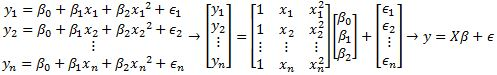Since we are minimizing the length of $\epsilon$, then we can find the least-squares solution $\beta$ using $X^T X\beta=X^T y$. This can also be applied to other functions.

Multiple Regression
Let the data points be $(u_1,v_1,y_1 ),(u_2,v_2,y_2 ),\cdots,,(u_n,v_n,y_n )$ and we want to use the best fit function $y=\beta_0+\beta_1 u+\beta_2 v$, where $\beta_0,\beta_1,\beta_2$ are parameters.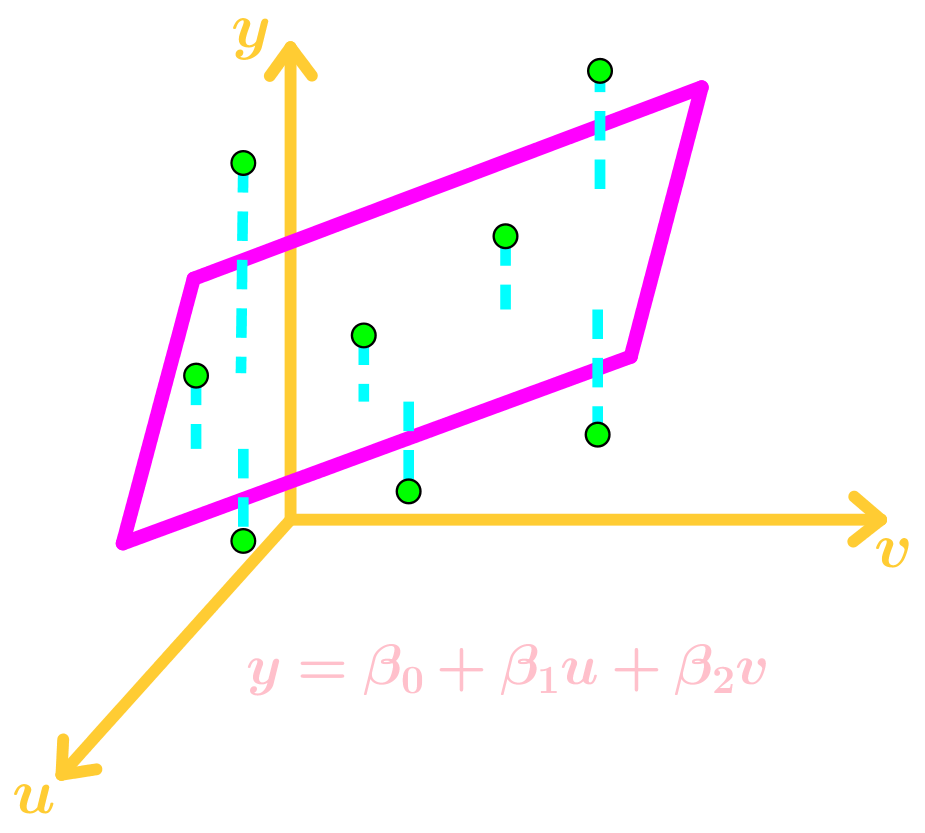Again, the data points don't actually lie on the function, so we add residue values $\epsilon_1,\epsilon_2,\cdots,\epsilon_n$ where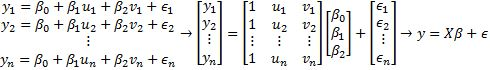Since we are minimizing the length of $\epsilon$, then we can find the least-squares solution $\beta$ using $X^T X\beta=X^T y$. This can also be applied to other multi-variable functions.